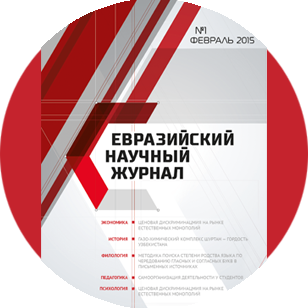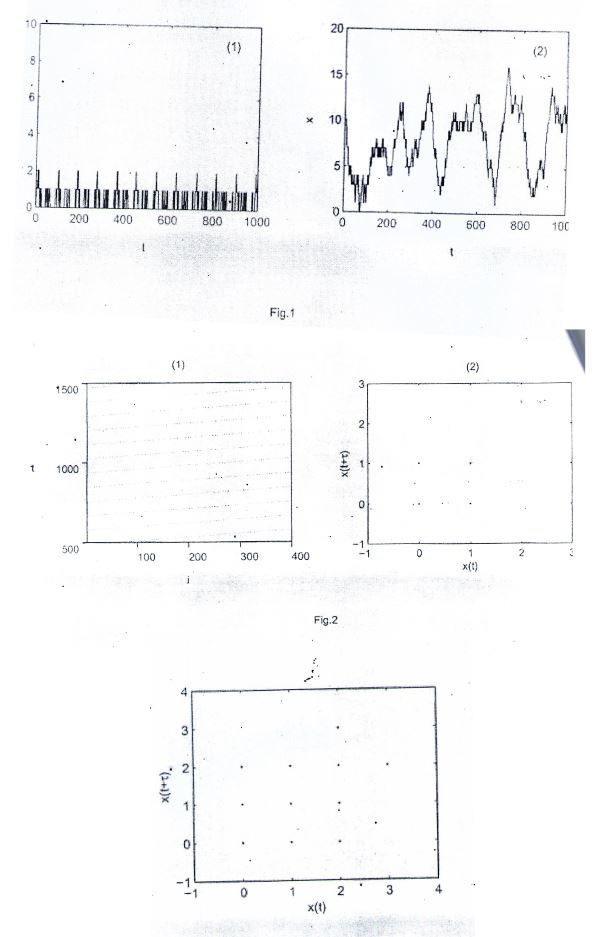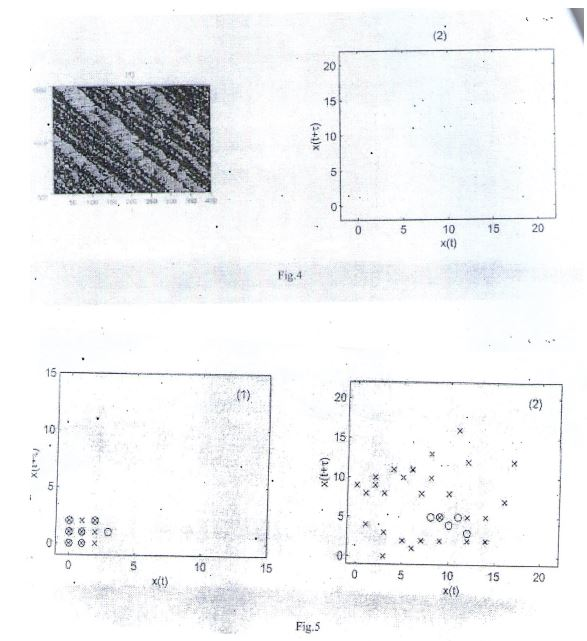Евразийский
научный
журнал

Срочная публикация научной статьи

+7 995 770 98 40
+7 995 202 54 42
info@journalpro.ru# The New Traffic Model With Dynamics Nature In ItПоделитесь статьей с друзьями:
Рубрика: Физико-математические науки
Журнал: «Евразийский Научный Журнал №9 2015»  (сентябрь 2015)
Количество просмотров статьи: 2951
The New Traffic Model With Dynamics Nature In It
Dr.Abdul Salaam .W.Alkhazraji
Faclty of Education
University of Garmian
Kurdistan Regional – Iraq

Abstract : In this paper I will discuss the two point following (Nasch Rraffic Model And Delay Reconstruction ) after then obtain the results The numerical simulation results demonstrate the existence of distinct dynamics states & numerous empirical data & anthers is the traffic flows shows various complex behavior . In order to obtain insight into the nature of dynamics , we apply the nonlinear time series analysis approach to study the characteristic behavior of traffic flow at low & intermediate density value. A procedure called embedding scheme has been to reconstruct the representation of the time series which is obtained from Nasch traffic model .We investigate the regular & the chaotic attractor of traffic time series in the reconstructed phase space. Our results indicate that nonlinear technique can be successfully used for understanding of dynamics feature of traffic flow .

Keywords : Delay reconstruction ; Nasch traffic model /: chaotic attractor.

Point one - Introduction

In the last decade been growing efforts made in the traffic models are proposed ]1[ and compared with real traffic date ]2[. With the these models, free flow and so-called wide traffic jams are well understood . On the hand the nature of congested traffic flow yet remains unclear despite various (3- 5)and theoretical efforts (6-9 . 10) . Becenl theoretical studies prose that gusted traffic flow may not be a single phase but rather a collection Of multiple each of which is realized under different conditions (8.9) . In the empirical investigate qualitatively distinct congested traffic states are reported (3.11)2

The complexity in traffic flow is linked to space time transitions between the traffic phase. An appearance of these phases is often accompanied by a very dynamical behavior of variables of the physical systems. An implicit but import ceptual implication of the recent theories has been discussed (6.9) where traffic are identified with resulting final states that traffic flow dynamics lead to after transient time, in the Language of nonlinear dynamics, all these states corresponding able attractors  of traffic flow dynamics. This concept mean that the results state is independent, of detail of the initial traffic state or its evolution history for external condition.

Chaotic phenomena in traffic models , has been reported. Addison and Low chaos in a single-lane car-following-model in which a leading car has oscillateity . Nagatani reported the presence of chaotic jam phase in a lattice hydra model.  model .L.A.Safonov el al. studied the presence of chaos in a ear- following model based on a system of delay-differential equations [l5]. In this paper, we used traffic model to simulate traffic flow, and capture the characteristic behavior of traffic flow. Our study is directed to investigate the dynamics character in phase analyzing the traffic time series, The paper is organized as follows; In .section 2. reduce the NaSch traffic model. The embedding scheme of lime series has been in section 3, and in Section 4 numerical and analytical results are presented conclusion of this approach Is presented.

Point two - NaSch Traffic Model

Nagel anil Srhreckenberg developed a one-dimensional probabilistic cellular Lion model  which is a model of traffic flow on a single-lane. The underlying of NaSch model is governed by the updated rules applied at. Discrete time step important features of traffic flow observed in real traffic can be well reproduced the model, such as the backward moving shock waves and phase separation. In Nagel and Schreckenberg model, the road consists of a single-lane which is into L cells of equal size numbered by i = 1,2….,L, and the time is discrete. 13 can be either empty or occupied by a vehicle with integer velocity v = 0, I……..i sites are simultaneously updated according to four successive Steps.

(1) Acceleration: increase U by 1 if U < u mnr

(2)Slowing down: decrease U to U = d if necessary (d is the number of empty. Front of the vehicle).

(3)Randomization» decrease U by I with randomization probability p if p >1

4) Movement: move vehicle' v sites forward.

Either period boundary condition or open boundary condition with. Nasch model is consider, In the case of period boundary condition , vehicles move and the global density is conserved quantity .system with open boundary can be characterized by the injection rate and the extinction rate . Most of for max > 1 assume period boundary condition (16.18) . The Nasch model is a model that all steps are necessary to reprodnce the hasic featues of real traffic investigations of traffic flow employing . Nasch traffic model have show that demonstrates complex phenomenal (1.19.20) . There types of traffic phase can be which are free flow , synchronized flow and jammed flow post is set to 20.The behavior of the time series X(t) is shown in Fig,1 at low density and inter density values. In Fig.(l), we can see that the occupation number of vehicle post is r (t) = 0 at some time. The result mean that, the traffic flow at low free flow, where the flux can be increased- by the increase of the density. The traffic corresponding to Fig .1 (2) is synchronized flow , where the density of vehicles than the corresponding values in free traffic. Using embedding method. We res the representation of time series x (t) in the phase space.

The vehicle density p is a important parameter. With the density p increas regular motion (i.e., fixed point, limit cycle) and haotic attractor can be observe reconstructed phase. In the reconstructed phase space, if the trajectory 1single fixed point , the trajectory state will remains unchanged as the time proceed viewed from the traffic model , the occupation number of vehicles on the proceed be unchanged for all time. This mean that site on the post, will always be full in the4 space-time diagrams. The instance can be found when the vehicle density at very low or high value.

When the density p is low, we can observe the limit cycle in the reconstruct space. In such case , the variation of time series X(t) is small and slow . Fig.2 the formation of small limit cycle with simple periodicity . In Fig.2. the Fig,2(1) space-time diagrams at low density p = 0.004. Accordingly, the reconstructed phase of the time series X (t) is placed in Fig.2(2) The traffic flows illustrated in Fig free flow with no jam (here, each dot corresponds to a vehicle). With the vehicle increased some other regular motions can be observed in the reconstructed phase Fig.3indicate this instance at density p - 0.04.

The attractor showed in Fig.3 finite points. This means that, all initial states will tend to periodic orbit on a results of simulation suggest that the trajectory illustrated in Fig.3 are to regular motion with multi-period. With further change of the density p. the traffic jam begin to emerge on the p representation of the embedding construction of the time series X(t) and the diagrams of the NaSch traffic mode! at intermediate density value are shown in Fig.1(1) the diagram shows the synchronized traffic flow with jams moving.

Were picture looks less ordered Fig. 4(2) exhibits the trajectories which dynamics behavior of the synchronized traffic flow in phase space. From Fig can see that the attractor is set of disperse points , and there are several it . This is the character of chaos . In the reconstructed phase spaces , we can as (local) Lyapunov exponent for each of the directions, and define a chaotic system a system which has at least one positive Lyapnnov exponent .Here, we calculate the largest Lyapnnov exponents of time series X (t) using the method (24) . The largest Lyapunov exponents time series X(t) using the method des?? (24) . The largest Lyapunov exponents of time seties X(t) using series X (t) obtained from Fig = 0.03, i.e > 0. The result means that the traffic flow showed in Chaotic.5

One of the features of deterministic chaos is sensitive to initial conditions. The dependence on initial conditions in the chaotic dynamics causes the nearby traffic dependence on initial conditions space. Fig.5 show what happens to small clouds diverge exponentially in phase space. Fig.5 show what happens to small clouds at nearby initial conditions in the reconstructed phase snace. Where each mark.

Point three - Delay Reconstruction

Embedding Scheme is one of the most important technical contribution of t?? of nonlinear dynamics , it has become the tool of choice in analyzing nonlinear.

W i t h the embedding method. -we can generalize the quantitative measures of behavior, Takins (21) shows that under suitable hypotheses, the trajectory in phase made from time series by embedding will have the same topological properties original attractor. Usually , if the original attractor has correlation dimension embedding dimension of +1 will be adequate for reconstructing the attractor?? . A standard procedure in the analysis of chaotic experimental data is to the attractor using the tim-delayed method. Suppose a time series ] [ t = is given using the method of delays , the Tata is represented in d￾dimensional vectors

Xt = ]Tt Tt + Tt T++(d-1)T [

where d is called the etrfbedding dimension and r is the delay –time. The recon attractor consists of points of the vector xt. Since the character of the overall of the stems is rejected in the behavior of any one dynamics variable, we cat that the dynamics of the vectors constructed in this way are the same as the trajectories in the full phase space of the system.6

The correlation dimension and the delay-time T are important parameter bedding scheme for example, if we make T too small, the total number of data required becomes very large, a suitable method used to choose the correlation d was address by Grassberger and Procaccia (22). The approximation of the time is equal to the time Is equal to the lag where the autocorrelation function drops to 1-1/e of its of its Calculating the autocorrelation function can be accomplished using the fast form (FFT).

The theoretical analysis of traffic jams has shown that important parameters jams (the velocity of a jam. the density and the flux of vehicles in the outfl￾ow jam, one of the phases of the traffic flow, appear as the density is larger than a critical value. As stay increases, the transition between free flow and jammed states can be observe has lea focus of traffic studies . These features of traffic jams also play an it role for other properties traffic (23) . In our approach, we choose the time series reflects the variation of the vehicle density on a given post.

Point four - The Results

We consider N vehicles moving on a road of L, sites. The boundary condition Nasch traffic model is periodic boundary. Since real traffic data can be well descries the parameters v, mn = 5 and p=0.5 (1), we assume these parameters in our pr. A small section of the road is designated as the observation post, which has a sites and M <

In Fig.5, each o marks an initial condition plotted as 1 Tt-T and the X marks the stale after iterating the dynamics of traffic flow for some marks . From Fig.5(1), we can find that the small clouds do not spread out after7 dynamics for one hundred steps. The results indicate that, the dynamical behave traffic How at low density value is the nonchabtic. In Fig.5(2), it is observed that.

Clouds broad over time after iterating the dvnamics for one hundred steps, where the nearby trajectories diverge exponentially. This means that, the dynamical be the traffic (flow is chaotic at intermediate densitv.

Conclusion

In conclusion, we use the embedding method to reconstruct traffic time series obtained from NaSch traffic model. The discussion in our reflect the local dynamical behavior of traffic flow. One of the important parameter M. The dynamical behaviors of the time series X(t) are mainly controlled by the length and site of the post. M. If we change the length and site of the post can obtain different attractor in phase space.

We increase the vehicle density p from low to intermediate value, and obtain dynamical behavior in the reconstructed phase space. When the. density p is can find the no chaotic attractor in the reconstructed phase space. With the increased, the traffic Jams begin to emerge. In this case, we can observe the attractor in phase space. In the framework of dynamical systems approach that of occupation on the post become large when a traffic jam appear, and the trajectory phase space expand in one direction. Construct in others, In practical problem develop models based on chaotic attractor to improve the prediction for traffic dis playing chaotic behavior, or to reduce the effects of noise in the time series date is obtained from traffic system.

References

1. K.Nagel and M.Schreckenberg Kenberg, j.Phys, I 2 . 2221(1992).
2. B.S Kerner H. Rehborn, Phys.Rev.E 53, Rl 297(1996).
3. B S Kb met and H.lic Hlom, Phvs.Rev E 5,3 R4275 (1996).
4. B.S Kemer and H.Rehborn, Phys.Rev.I.er.r. 79. 4030(1997).
5. B.S.Kcmer, Phys.RevLett. 81, 1130(1998).
6. II.Y.Lee, H.-w. Lee, and D.Kim. Phys.Rev.Lett. 81, 1130(1998).
7. D.Helbing M.Treiber. Phys.Rev.Lctt. 81, 3042(112(1998).
8. D.Helbing. A.Hennecke and M.Treiber,phys.Rev.Latt .82,4360(1990).
9. H.Y.Lee. II. W T.Ee. and D Kim, Phys.Rev.B 59, 5101(1999).
10. E.Tomer, L.Safonov, and S.Harlin, Phys.Rev.lett. 84. 382(2000).
11. H.Y.Lee, H.W. Lee, and D.Kim, phys.E 62. 4737(2000).
12. E.A.Jackson, Perspectives Of Nonlinear Dynamics Cambridge University Pre. bridge, 1989), Vol. 1.
13. Addison PS. And Low D.J.. Chaos. 8. 791(1998).
14. Nagatani T., Phys.Rev.E- 60. 1535(1999)
15. L.A.Snfonov. E Tomer, Y.V.St rygrn. V. Aahkenazy and S.Havlin, Furopb 57(2), 151 (2002),
16. L.C.Q. Vilar and A.M.C. De Souza, Physira A 211, 84(19941.
18. A.Schadschneider and M.Sehrekenberg. J.Phys.A 31. 1.225(1998).
19. MSchreckenberg, A.Schadschneider, k.Nagel, and N Ito. Phys.Rev.E 51, 29:9
20. D.Chowdhury. L.Santen, and A.Schadschneider, Phys.Rep. 329. 1999(2000).
21. F. Takens, Lecture Notes in Mathematics Vol. 898 Springer, New York. 198.
22. P.Grassberger and I.Procaccia, Physica D 9. 189(1983).
23. B.S.Keme and P.Konhauser, Phys.Rev,E 50. 54(1994).
24. Rosenstein M.T., Collins J.I. and De Luca C. J.,Physica D 65. 117(1993).

Figure

• Figure I Time series X (t) at low density p = 0.02 (1) and intermediate density (2),
• Figure 2 (1) Space-time diagram of the traffic flow for P 0.004. (2) the stracted Phase space of the time series X(t) for P = 0.004 t = 4.
• Figer 3 The reconstructed phase space of the time series X(t) for p =0.04 T =
• Figure 4 (1) Space-time diagram of the traffic flow for p = 0.4 (2) The strueted; Phase a space of the time scries .X (t) for p = 0.4, - T=17.
• Figure 5 Schematic diagram of diverging initial conditions for p
=0.02,T=5
p = 0.4 T= 17 (2),
••